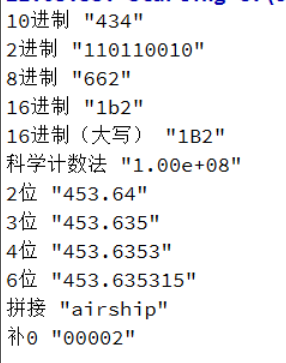• QString QString::number(long n, int base = 10) 返回一个包含该字符串的n个字符的字符串，从指定的位置索引开始。 如果位置索引超过字符串长度，则返回空字符串。如果从给定位置开始的字符串中可用的字符少于n个，...
文章介绍

Qt提供非常多使用的工具类，如排序、类型转换、格式转换等。很基础的工具类都需要查看文档或者百度。做个记录笔记，增加印象同时方便查阅。~
函数重载：常见的QString的转换

//用来做进制的转换
qDebug() << "10进制" << QString::number(src_a,10);
qDebug() << "2进制" << QString::number(src_a,2);
qDebug() << "8进制" << QString::number(src_a,8);
qDebug() << "16进制" << QString::number(src_a,16);
qDebug() << "16进制（大写）" << QString::number(src_a,16).toUpper();

//科学计数法
long src_e = 100000000;
qDebug() << "科学计数法" << QString::number(src_e,'e',2);

//控制输出的位数
float src_b = 453.6353;
qDebug() << "2位" << QString::number(src_b,'f',2);
qDebug() << "3位" << QString::number(src_b,'f',3);
qDebug() << "4位" << QString::number(src_b,'f',4);
qDebug() << "6位" << QString::number(src_b,'f',6);

//字符串拼接(放在前面)
QString x = "ship";
QString y = "air";
qDebug() << "拼接" << x.prepend(y);

//前面补0
qDebug() << "补0" << QString::number(2).asprintf("%05d", 2);

结果展开全文qt5
• QString s = QString::number(a, 16); // s == "3f" QString t = QString::number(a, 16).toUpper(); // t == "3F" //支持格式:long, int, uint, ulong, qlonglong, quint8,quint16,quint32 //转10进制就讲16改为...

long a = 63;
QString s = QString::number(a, 16);             // s == "3f"
QString t = QString::number(a, 16).toUpper();     // t == "3F"

//支持格式:long, int, uint, ulong, qlonglong, quint8,quint16,quint32
//转10进制就讲16改为10, 支持2-36进制转换

展开全文qt c++
• QString::number()

千次阅读 2020-12-16 15:45:11
QString::number是将数数字（整数、浮点数、有符号、无符号等）转换为QString类型，常用于UI数据显示。查看API接口，number提供如下几种类型的转换，适合各种场合调用。 static QString number(int, int base=10)

1.写在前面

Qt提供非常多使用的工具类，如排序、类型转换、格式转换等。如果是经常使用则信手拈来，否则间隔时间长了，很基础的工具类都需要查看文档或者百度。所以做个言简意赅的记录笔记，增加印象同时方便查阅。

2.QString::number

QString::number是将数数字（整数、浮点数、有符号、无符号等）转换为QString类型，常用于UI数据显示。查看API接口，number提供如下几种类型的转换，适合各种场合调用。

static QString number(int, int base=10);
static QString number(uint, int base=10);
static QString number(long, int base=10);
static QString number(ulong, int base=10);
static QString number(qlonglong, int base=10);
static QString number(qulonglong, int base=10);
static QString number(double, char f='g', int prec=6);

第一个参数：待转换数字

第二个参数（整型）：转换进制

第二个参数（浮点数）：浮点数格式

第三个参数（浮点数）：保留小数位数

默认情况下是十进制显示方式转换，也可以使用八进制、十六进制显示方式调用。

3.QString::number 整数转换

int a = 20;
uint b =255;
QString::number(a);
QString::number(a,10);
QString::number(b);
QString::number(b,16);

结果：“20”，“20”，“255”，“ff”。

4.QString::number 浮点数转换

1)保留2位小数

float a;
QString::number(a,‘f’, 2);

例如：

1.234，结果：1.23

1，结果：1.00

2)保留2位有效数字，以简单方式表示，或者科学计数法表示

float a;
QString::number(a, ‘g’, 2);

例如：

0.00001，结果：1e-5

0.00012，结果：1.2e-4

1.23，结果：1.2

3)保留指定位数（如001）

整数转换为字符串，并保留3位，不足在前面补0，这一块其实属于“sprintf”格式函数的范畴：

int a=1;
QString str=QString::number(a).sprintf("%03d",a);
//或者
QString str=QString("%1").arg(a, 3, 10, QChar('0'));

展开全文• qstring::number的使用

千次阅读 2019-12-27 20:46:54
近期进行Qt的一些编程的时候出现格式的Qstring的格式问题 小数点后的位数 如下 QString::number(value,'f',3) 1 这个是小数点后有三位 float型。后面用到的比较多 ...

近期进行Qt的一些编程的时候出现格式的Qstring的格式问题
小数点后的位数
如下

QString::number(value,'f',3)

• 1

这个是小数点后有三位 float型。后面用到的比较多

展开全文• QString::number 方法可以用于位数的保留： 例如 QString::number(str, ‘f’, 2); // 保留2位小数，例如 1.234->1.23 1->1.00 2.1023->2.10 QString::number(str, ‘g’, 2); 保留2位有效数字，并采用...
• QString::number

2021-02-26 10:46:47
int a = 1024; QString::number(a);//1024
• 在Qt5.12Creator编译环境中，int变量转QString变量的一个方法是用QString::number函数来转换，具体的用法如下所示： 1.1QString::number示例用法 quint32MAC=255; QStringMac1=QString::number(MAC,10);//第一个...
• 1、 QString::number(average, 'f', 5)，这里的'f'表示的是：‘f’方式结果是0.00，所以后面的5表示的就是输出的时候保留5位小数 2、这种的QString::number(average, ‘g’, 3); ，这里的'g'表示的是：’g’方式...
• 在Qt5.9Creator4.4.1编译环境中，int变量转QString变量的一个方法是用QString::number函数来转换，具体的用法如下所示： 1.1QString::number示例用法 quint32 MAC = 255; QString Mac1 = QString::number...Qt5.9 int QString Qt基本数据类型
• QString::number 保留2位小数

千次阅读 2018-02-24 21:48:46
QString::number(num, 'f', 2);保留小数
• Qt 学习笔记 - QString::number

千次阅读 2013-03-28 22:48:21
QString QString::number ( long n, int base = 10 ) [static] 主要作用是将n转化为字符串。 参数base表示将n转化为字符串时，是以多少进制为参照的。默认情况下是以十进制为参照进行转化的。base范围必须在2 - ...Qt
• QString strTmp = "test";const char *pTmp = QString::toStdString().c_str();今天调试程序出现pTmp指向字符串为空，当时分析是临时变量销毁了std::string s = strTmp.toStdString(); const char* pTmp ...
•  QString tem = QString("%1,%2,%3,%4,%5").arg(thr1, 3, 10, QChar('0')).arg(thr2,3, 10, QChar('0')).arg(thr3, 3, 10, QChar('0')).arg(thr4, 3, 10, QChar('0')).arg(thr5, 3, 10, QChar
• QString类—number Acuity. 2018-01-04 17:13:25 8717 已收藏 24 展开 1.写在前面 Qt提供非常多使用的工具类，如排序、类型转换、格式转换等。如果是经常使用则信手拈来，否则间隔时间长了，很基础的工具类都需要...
• QStringList QString::split(const QString &amp;amp;amp;sep, SplitBehavior behavior = KeepEmptyParts, Qt::CaseSensitivity cs = Qt::CaseSensitive) const Splits the string into ...
• QString是Qt中使用频率最高的几种数据类型之一，主要在于其提供了大量功能强大的成员，其中有几个方便的操作与大家分享一下： 1.字符串处理与匹配 （1）mid()：返回给定位置（参1）到给定长度（参2）的子串 这是...C++ 容器 qt QString 数据
• QString

2020-11-08 20:43:59
asprintf 从格式字符串cformat和任意参数列表安全地构建格式化字符串 m_labCellPos->setText(QString::asprintf(“当前单元格：%d行, %d列”, current.row(), current.column()));
• Qt：关于QString索引为负数的一些发现 使用QString截取字符串，调用mid函数，str2.mid(-5,3)，发现返回为空字符串，后来想起原来是python可以肆意使用负数索引。但又发现之前使用remove函数成功的删除了一段字符串。...字符串 编程语言
• 1、QString格式化arg前面自动补0 int seconds = m_calc.elapsed() / 1000; int hour = int(seconds / 3600); int min = int((seconds - hour * 3600) / 60); int sec = seconds % 60; //QString格式化arg前面自动...QString 格式化
• 一、QString的size()与QByteArray的size()的区别 在实际测试中，发现QByteArray的size与QString的size不一致 原因是，报文中打包的是通过QByteArray::size()算出的大小，而解包的时候却是用的QString::size()算出的...
• Qt QString类 常用方法

万次阅读 2018-04-25 10:44:37
QString类（常用函数） QString类提供了一个Unicode文本和经典的C以零结尾的字符数组的抽象。 （1）QString::QString() 构造一个零字符串。这是一个不被分配任何东西的字符串，也就是说长度和数据指针都为0。如何...
• ui->lin3->setText(QString::number(sum)); 个人总结： 不能太相信老师所提的，所以还是要自己学习，QT的ui界面创建比无ui界面的要简单，所以时间允许的话，可以再考虑考虑硬编码，锻炼一下自己的代码能力、...qt 学习总结 代码
• QString是Qt中使用频率最高的几种数据类型之一，主要在于其提供了大量功能强大的成员函数，这里重点介绍一些常用的成员函数： 一、字符串处理相关 1.1 split() （拆分字符串） split() 函数可以将一个字符串，拆分......